# Quadratic Equations: College Precalculus Lesson Plans Chapter Exam

Exam Instructions:

Choose your answers to the questions and click 'Next' to see the next set of questions. You can skip questions if you would like and come back to them later with the yellow "Go To First Skipped Question" button. When you have completed the practice exam, a green submit button will appear. Click it to see your results. Good luck!

### Page 1

#### Question 1 1. If the equation below told you a ball was 'h' feet in the air after 't' seconds, what would be the first step to finding out when it hit the ground?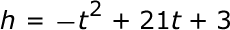#### Question 2 2. Use the quadratic formula to find the solutions to the equation below.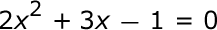### Page 2

#### Question 6 6. Find the center and radius of the following equation: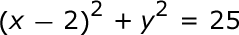#### Question 7 7. Rewrite the quadratic below in standard form: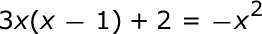#### Question 8 8. True or False: This is the graph of a function.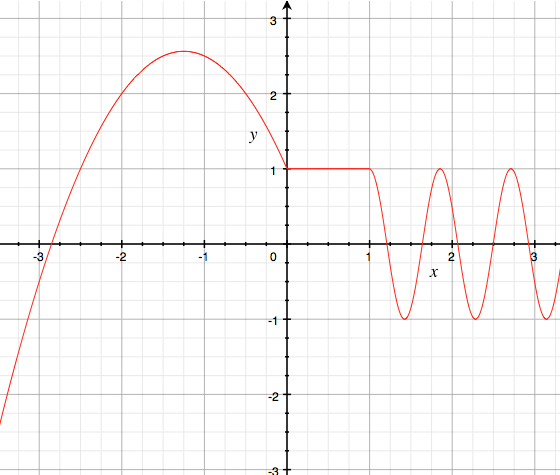### Page 3

#### Question 11 11. Rewrite the following equation in the vertex form.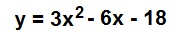#### Question 12 12. Solve the equation below.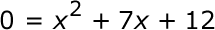#### Question 13 13. When completing the square on the quadratic equation listed below, how is c calculated?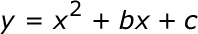#### Question 14 14. What would the first step be in completing the square of the following equation?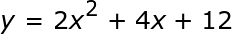#### Question 15 15. Solve the equation below.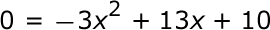### Page 4

#### Question 19 19. Find the center and radius of the following equation: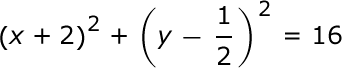#### Question 20 20. In order to complete the square on the following equation, what value would you add to both sides?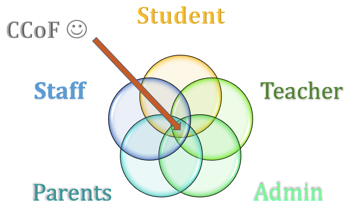### Page 5

#### Question 25 25. Find the roots of the equation.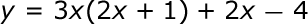### Page 6

#### Question 27 27. True or False: This is the graph of a function.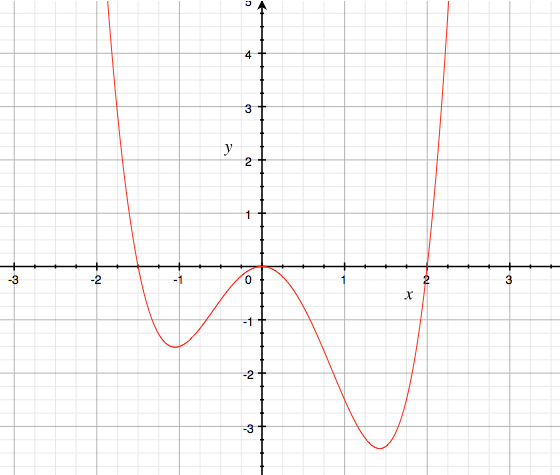#### Question 28 28. What is the b-value of the quadratic equation below?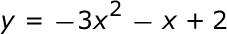#### Question 29 29. Solve the equation.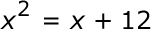#### Quadratic Equations: College Precalculus Lesson Plans Chapter Exam Instructions

Choose your answers to the questions and click 'Next' to see the next set of questions. You can skip questions if you would like and come back to them later with the yellow "Go To First Skipped Question" button. When you have completed the practice exam, a green submit button will appear. Click it to see your results. Good luck!

Support